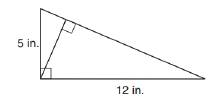Chapter 8.1, Problem 47E### Elementary Geometry for College St...

6th Edition
Daniel C. Alexander + 1 other
ISBN: 9781285195698

#### Solutions

Chapter
Section### Elementary Geometry for College St...

6th Edition
Daniel C. Alexander + 1 other
ISBN: 9781285195698
Textbook Problem
4 views

# In Exercises 47 to 50, use the fact that the area of the polygon is unique.In the right triangle, find the length of the altitude drawn to the hypotenuse.To determine

To find:

The length of the altitude drawn to the hypotenuse.

Explanation

Converting theoretical statement into mathematical statement, identifying the geometric figure and the calculation is done by suitable geometric formulae.

Calculation:

Given,

The lengths of the sides are,

AB=a=5 in

BC=b=12 in

Area of the right ΔABC(A)=12ab unit2.

=12(5)(12)

=(5)(6)

A=30 in2

Find AC:

For right triangle ABC

Here AB=5 in and BC=12 in

By Pythagorean theorem,

(AB)2+(BC)2=(AC)2

(5)2+(12)2=(AC)2

### Still sussing out bartleby?

Check out a sample textbook solution.

See a sample solution

#### The Solution to Your Study Problems

Bartleby provides explanations to thousands of textbook problems written by our experts, many with advanced degrees!

Get Started

#### Find the distance between (2, 4) and (6, 8).

Applied Calculus for the Managerial, Life, and Social Sciences: A Brief Approach

#### The graph of f(x) = 3x5 − 5x3 is:

Study Guide for Stewart's Single Variable Calculus: Early Transcendentals, 8th

#### True or False: is a convergent series.

Study Guide for Stewart's Multivariable Calculus, 8th